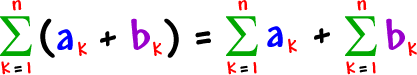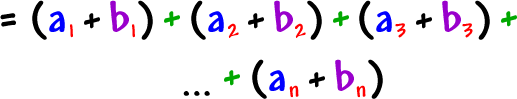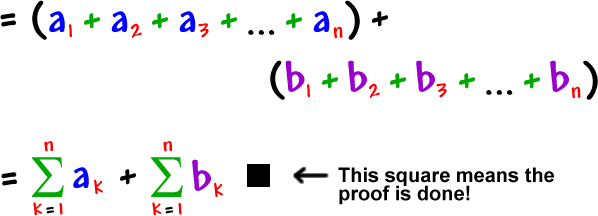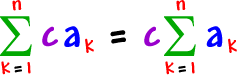If you're going on to Calculus, you're going to need these!

Here's the first one:Here's an example so you can believe it:

Find this sum:Now, find these sums and add them:Do you believe it?  (You should have gotten the same thing for both.)

This may not seem like a big deal, but you'll need it to prove some important calculus stuff, so let's get used to it.

Here's the proof:Just expand it out...Now, use the commutative and associative properties...  Gather up the a's...  Gather up the b's...Here are the other two properties -- I'll let you do the proofs:of 1